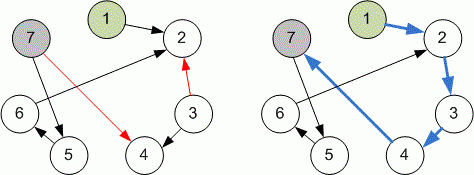favorite We need a little bit of your help to keep things running, click on this banner to learn more
Problems

# Reverse the graph

You are given a directed graph with n vertices and m edges. The vertices in the graph are numbered from 1 to n. What is the minimum number of edges you need to reverse in order to have at least one path from vertex 1 to vertex n.

#### Input

First line contains two integers n and m (1n, m2 * 106) - the number of vertices and the number of edges. The i-th line of the next m lines contains two integers xi and yi (1xi, yin), denoting that the i-th directed edge connects vertices from xi to yi.

#### Output

Print the minimum number of edges we need to invert. If there is no way of having at least one path from 1 to n, print -1.Time limit 1 second
Memory limit 128 MiB
Input example #1
7 7
1 2
3 2
3 4
7 4
6 2
5 6
7 5

Output example #1
2